GFG App
Open AppBrowser
Continue

# Types of Frequency Distribution

It is not always possible for an investigator to easily measure the items of a series or set of data. To make the data simple and easy to read and analyze, the items of the series are placed within a range of values or limits. In other words, the given raw set of data is categorized into different classes with a range, known as Class Intervals. Every item of the given series is put against a class interval with the help of tally bars. The number of items occurring in the specific range or class interval is shown under Frequency against that particular class range to which the item belongs.

#### Example,

The marks of a class of 20 students are  11, 27, 18, 14, 28, 18, 2, 22, 11, 24, 22, 11, 8, 20, 25, 28, 30, 12, 11, 8. Prepare a frequency distribution table for the same.

#### Solution:

The range of marks of the students is 2- 28. Let us take class intervals 0-5, 5-10, 10-15, 15-20, 20-25, and 25-30.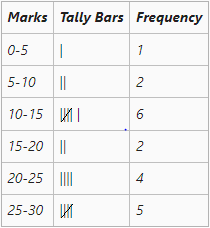## Types of Frequency Distribution

The six different types of the frequency distribution are as follows:

1. Exclusive Series
2. Inclusive Series
3. Open End Series
4. Cumulative Frequency Series
5. Mid-Value Frequency Series
6. Equal and Unequal Class Interval Series

### 1. Exclusive Series

The series with class intervals, in which all the items having the range from the lower limit to the value just below its upper limit are included, is known as the Exclusive Series. This kind of frequency distribution is known as exclusive series because the frequencies corresponding to the specific class interval do not include the value of its upper limit. For example, if a class interval is 0-10, and the values of the given series are 4, 10, 2, 15, 8, and 9, then only 4, 2, 8, and 9 will be included in the 0-10 class interval. 10 and 15 will be included in the next class interval, i.e., 10-20. Also, the upper limit of a class interval is the lower limit of the next class interval.

#### Example,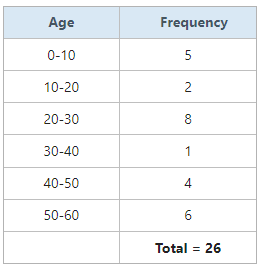From the above table of exclusive series, it can be seen that the upper limits of the first class interval is the lower limit of the second class interval, and so on. Also, as discussed above, if the data includes a value 10, it will be included in the class interval 10-20, not in 0-10.

### 2. Inclusive Series

The series with class intervals, in which all the items having the range from the lower limit up to upper limit are included, is known as Inclusive Series. Like exclusive series, the upper limit of one class interval does not repeat itself as the lower limit of the next class interval. Therefore, there is gap (between 0.1 to 1) between the upper class limit of one class interval and the lower limit of the next class interval. For example, class intervals of an inclusive series can be, 0-9, 10-19, 20-29, 30-39, and so on. In this case, the gap between the upper limit of one class interval and the lower limit of the next class interval is 1, and the class intervals do not overlap with each other like in exclusive series.

Sometimes it gets difficult to perform statistical analysis with inclusive series. In those cases, the inclusive series is converted into exclusive series.

#### Example,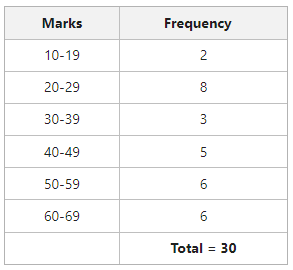From the above table of inclusive series, it can be seen that the upper limit of one class interval (say, 9 of interval 0-9) is not the same as the lower limit of the next class interval (10 of interval 10-19). Also, all the values that come under 0-9, including 0 and 9 are included in the frequency against 0-9.

#### Conversion of Inclusive Series into Exclusive Series

For statistical calculation, sometimes it becomes necessary to convert the inclusive series into exclusive series. Suppose, in the above example some students have obtained marks such as 10.5, 40,5, etc. In this case, this series will be converted into exclusive series,

The steps for converting an inclusive series into exclusive series are:

• In this first step, calculate the difference between the upper class limit of one class interval and the lower limit of the next class interval.
• The next step is to divide the difference by two and then add the resulting value to the upper limit of every class interval and subtract it from the lower limit of every class interval.

#### Example,

The inclusive series of the above example is converted into exclusive series as under.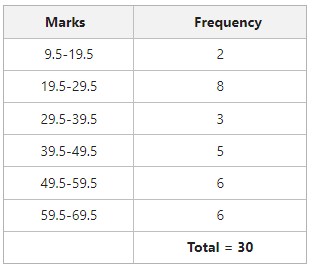#### Difference between Inclusive and Exclusive Series

• In Inclusive Series, the upper limit of one class interval is not the same as the lower limit of the next class interval. There is a gap ranging from 0.1 to 1.0 between the upper class limit of one class interval and the lower class limit of the next class interval. However, in the Exclusive Series, the upper limit of one class interval is the same as the lower limit of the next class interval.
• In the case of Inclusive Series, the value of the upper and the lower limit are included in that class interval only. However, in the case of Exclusive Series, the value of upper limit of a class interval is not included in that interval, instead, it is included in the next class interval.
• Inclusive Series is suitable for an investigator only if the value is in complete number and not in decimal form. However, an Exclusive Series is suitable for an investigator whether the value is in complete number or decimal form.
• Counting in Inclusive Series is possible only after converting it into an Exclusive Series. However, counting in Exclusive Series is possible in all cases.

### 3. Open End Series

Sometimes the lower limit of the first class interval and the upper class limit of a series is not available; instead, Less than or Below is mentioned in the former case (in place of the lower limit of the first class interval), and More than or Above is mentioned in the latter case (in place of the upper limit of the last class interval). These types of series are known as Open End Series.

#### Example,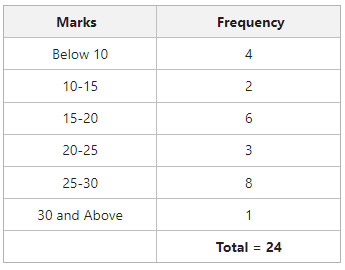For statistical calculations, if one needs to change the first and last class open-end class interval into limits, it can be done by the general practice of giving the same magnitude or class size to these intervals as the class size of other class intervals. In the above example, the magnitude of other class intervals is 5. Therefore, the open-end class intervals can be written as 5-10 and 30-35, respectively.

### 4. Cumulative Frequency Series

A series whose frequencies are continuously added corresponding to the class intervals, is known as Cumulative Frequency Series.

#### Conversion of a Simple Frequency Series into Cumulative Frequency Series

A simple frequency series can be converted into a cumulative frequency series. There are two ways through which it can be done. These are as follows:

• Expressing the cumulative frequencies on the basis of upper limits of the class intervals. For example, expressing 10-20, 20-30, and 30-40 as Less than 20, Less than 30, and Less than 40.
• Expressing the cumulative frequencies on the basis of lower limits of the class intervals. For example, expressing 10-20, 20-30, and 30-40 as More than 20, More than 30, and More than 40.

#### Example,

Convert the following simple frequency series into a cumulative frequency series using both ways.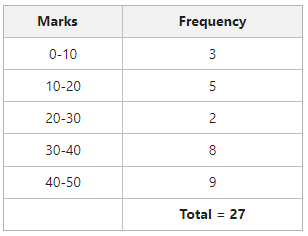#### Solution:

Method-I (On the Basis of Upper Limits)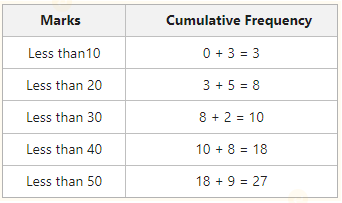Method – II (On the Basis of Lower Limits)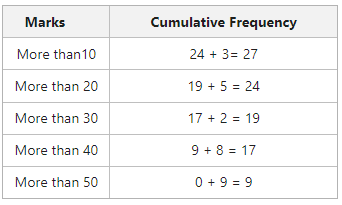#### Conversion of Cumulative Frequency into Simple Frequency Series

To attain the frequency against a specific class interval of a cumulative frequency series, it can be converted into a simple frequency series.

#### Example,

Determine the frequency of the following cumulative frequency series.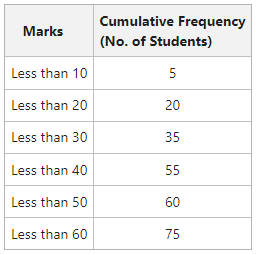#### Solution: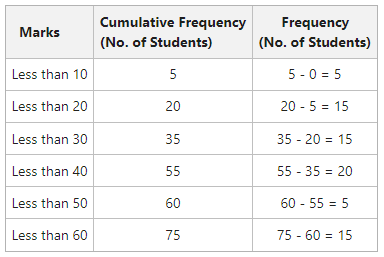### 5. Mid-Value Frequency Series

The series in which, instead of class intervals, their mid-values are given with the corresponding frequencies, is known as Mid-Value Frequency Series.

#### Conversion of Mid-Value Frequency Series into Simple Frequency Series

The steps to convert a mid-value frequency series into a simple frequency series are as follows:

• The first step is to determine the mutual difference between the mid-values.
• The next step is to obtain half of the resulting difference.
• The last step of conversion is to subtract the resulting figure from the second step from the mid-value to get the lower limit of the class interval, and add the resulting figure from the second step to the mid-value to get the upper limit.m = Mid-Value

i = Difference between mid-values#### Example,

Convert the following Mid-Value Frequency Series into Simple Frequency Series.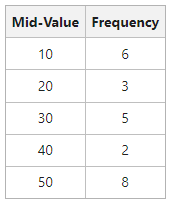#### Solution: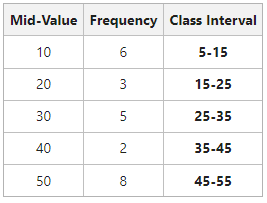#### Calculation:

Difference between mid-values (i) = 10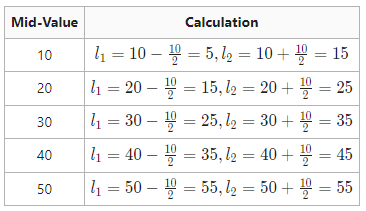### 6. Equal and Unequal Class Interval Series

#### Equal Class Interval Series:

When the classes of a series are of the same interval, it is known as Equal Class Interval Series.

#### Example,

Following is the frequency distribution of marks of 25 students with equal class intervals.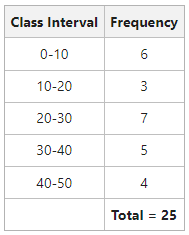#### Unequal Class Interval Series:

When the classes of a series are of unequal interval, it is known as Equal Class Interval Series.

#### Example,

Following is the frequency distribution of marks of 30 students with unequal class intervals.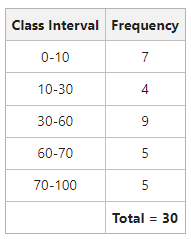My Personal Notes arrow_drop_up